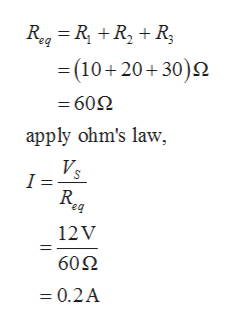# R1 100R2 20Vs 12vR3 300Во

Question

For the series circuit shown, what is the voltage drop across each resistor?What is the current through each resistor?

check_circleExpert Solution
Step 1

In the given circuit, all the resistors are in series. Hence, the current will be the same in each resistor.

Step 2

The current in the circuit is ...help_outlineImage TranscriptioncloseReg=R RR (10+20+30) = 602 apply ohm's law, Vs I = R eq 12V 60Ω =0.2A fullscreen

### Want to see the full answer?

See Solution

#### Want to see this answer and more?

Solutions are written by subject experts who are available 24/7. Questions are typically answered within 1 hour*

See Solution
*Response times may vary by subject and question
Tagged in

### Electrical Engineering